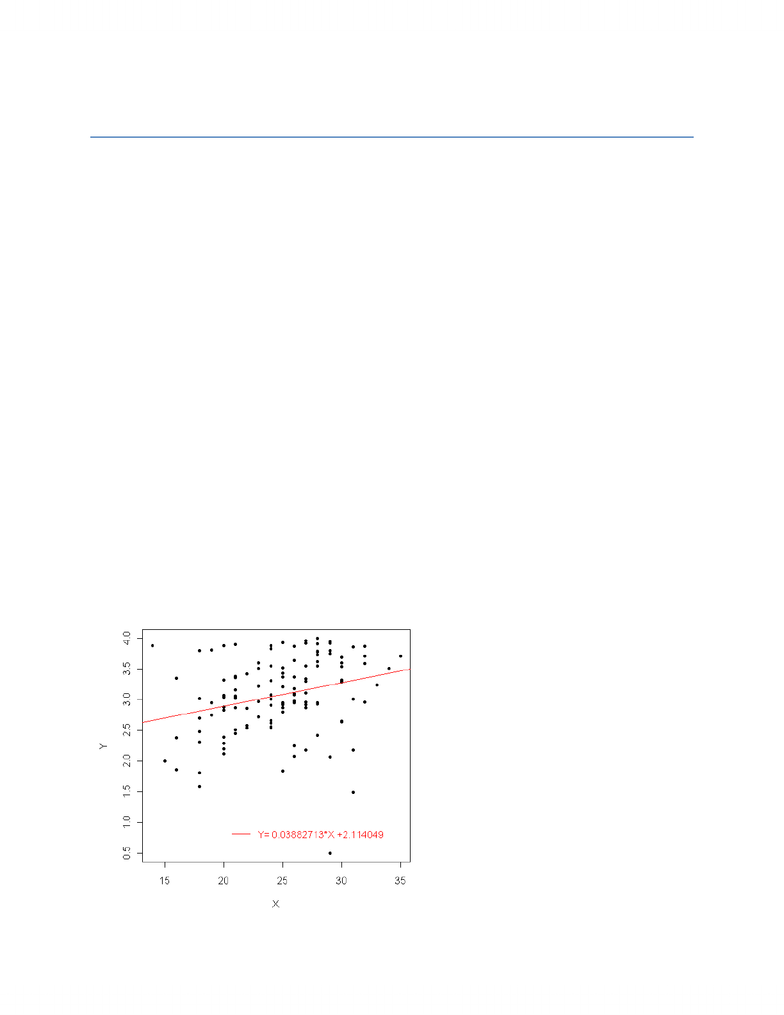Class Notes (1,100,000)
CA (620,000)
UTSC (30,000)
Lecture

STAC67H3 Lecture Notes - .De, Confidence And Prediction Bands, Point Estimation

Department
Statistics
Course Code
STAC67H3
Professor
R.Salakhutdinov

This preview shows pages 1-2. to view the full 7 pages of the document.AMS578Homework1solution
Problem 1:
a.
> setwd("C:/spring 2009 TA/hw1");
> file<-"CH01PR19.txt";
> attach(data);
> muy<-mean(Y);
> mux<-mean(X);
> b1<-sum((X-mux)*(Y-muy))/sum((X-mux)^2);
> b0<-muy-b1*mux;
> b1
 0.03882713
> b0
 2.114049
So the LSE of β0 and β1 are, separately, 2.114049 and 0.03882713.
The estimated regression function is: Y= 0.03882713*X +2.114049
b.
> par(mar<-c(4,4,1,1));
NULL
> plot(Y~X, pch=20);
> abline(b0,b1,col=2);
> legend(20,1, legend=c("Y= 0.03882713*X +2.114049"), col=2,lty=1,text.col=2,bty="n");

Only pages 1-2 are available for preview. Some parts have been intentionally blurred.c.
E(Y|X= 30) = 0.03882713*30 +2.114049 = 3.278863
d.
The point estimate of the change in the mean response is just the b1, 0.03882713.
Problem 2:
a.
> resid<-Y-(0.03882713*X +2.114049);
> MSE<-sum(resid^2)/118;
> sb1<-sqrt(MSE/sum((X-mux)^2));
> sb1
 0.01277302
> CI<-c(b1-qt(0.995,118)*sb1,b1+qt(0.995,118)*sb1)
> CI
 0.005385614 0.072268640
So the 99 percent confidence interval is (0.005385614, 0.072268640), which doesn’t include
zero. That means there is the significant relationship existed between the ACT score and the
GPA at the end of the freshman year.
b.
H0: β1= 0;
Ha: β1 0;
And the decision rule is if |t*| t1-a/2,n-2, We will reject H0.
Here a=0.01, n= 120 So:
> ts=b1/sb1;
> abs(ts)-qt(0.995,118);
 0.4216398
That means |t*| t0.995,118, which means given the significance level 0.01, we reject H0.
c.
> pvalue<-2*pt(-ts,118);
> pvalue;
 0.002916604
The P-value is 0.002916604, less than 0.01. So it gives the same decision in part (b).
Problem 3:
a.
> Yh=b0+b1*28;
> sYh=sqrt(MSE*(1/120+sum((28-mux)^2)/sum((X-mux)^2)));
> YhCI<-c(Yh-qt(0.975,118)*sYh,Yh+qt(0.975,118)*sYh);
> YhCI
You're Reading a Preview

Unlock to view full version